Learning Library

# Colour by Number Subtraction

Help make two-digit subtraction a little more colorful for your second grader with these colour by number worksheets.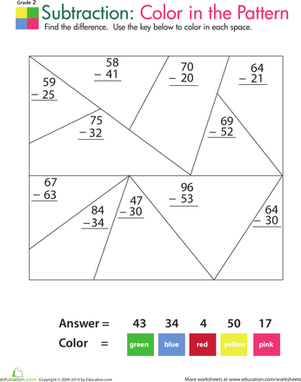### Colour by Number: practise Two-Digit Subtraction 1

Is your child tired of the usual maths worksheets? Modeled after classic colour by number pages, this subtraction worksheet makes finding the difference fun!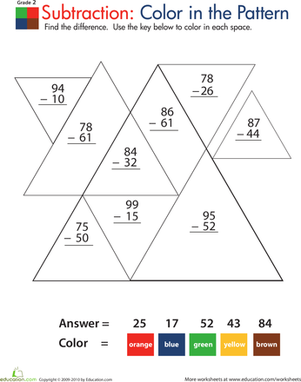### Colour by Number: practise Two-Digit Subtraction 2

Modeled after colour by number coloring pages, this maths worksheet makes subtraction fun! Your child will enjoy coloring to reveal a pretty geometric pattern.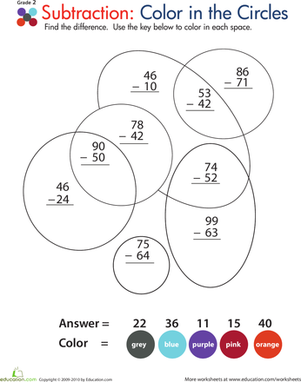### Colour by Number: practise Two-Digit Subtraction 3

Part colour by number coloring page, part maths worksheet, this year three printable have your child begging to practise subtraction!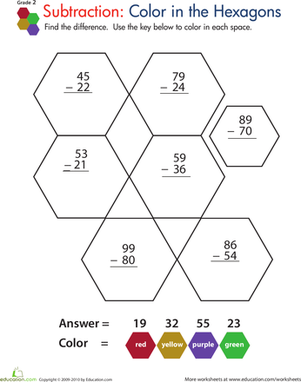### Colour by Number: practise Two-Digit Subtraction 4

Subtraction is fun in this year three maths worksheet modeled after classic colour by number pages.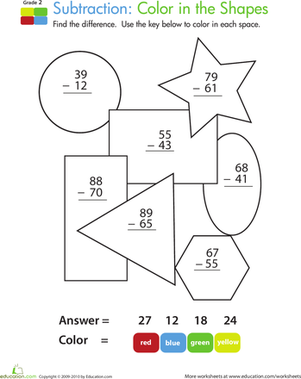### Colour by Number: practise Two-Digit Subtraction 5

Doubling as a colour by number coloring page, this year three maths worksheet makes practising two-digit subtraction a blast!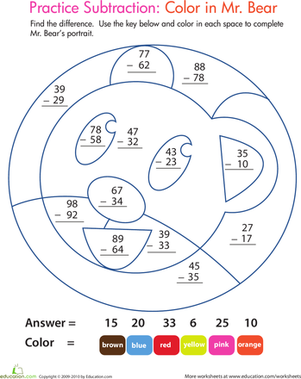### Colour by Number: practise Two-Digit Subtraction 6

This maths worksheet is just the thing to entice your child to practise subtraction. Once he's solved the two-digit subtraction problems, he'll colour Mr. Bear.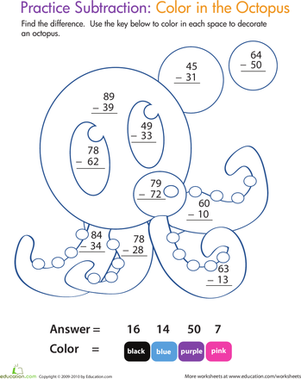### Colour by Number: practise Two-Digit Subtraction 7

Doubling as a colour by number coloring page, this year three maths worksheet makes practising two-digit subtraction fun and enriching at the same time!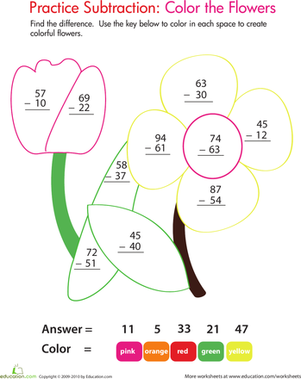### Colour by Number: practise Two-Digit Subtraction 8

This subtraction colour by number worksheet offers both maths enrichment and a coloring activity. Second graders solve two-digit problems, then colour!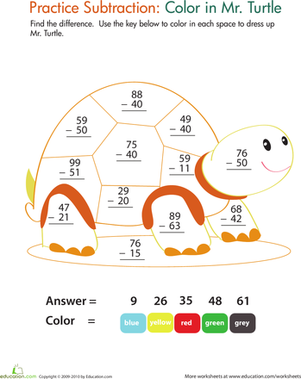### Colour by Number: practise Two-Digit Subtraction 9

Doubling as a colour by number coloring page, this year 3 worksheet makes practising two-digit subtraction fun without skimping on the maths help.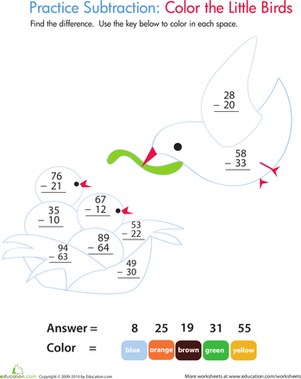### Colour by Number: practise Two-Digit Subtraction 10

Doubling as a colour by number coloring page, this year 3 maths worksheet makes practising two-digit subtraction fun.

Create new collection

0

### New Collection>

0Items

What could we do to improve Education.com?